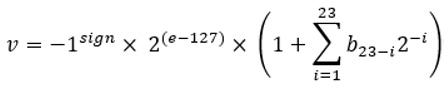# Chapter 9: Bit Manipulation

• 您必须非常了解位理论（例如，位运算符）
• 你必须尽可能多地练习位操作

• 了解位操作
• 编码挑战

# Bit manipulation in a nutshell

• 51的二进制表示是110011。
• 因为 51 是 `int`，所以表示为 32 位值；即 1 或 0 的 32 个值（从 0 到 31）。
• 110011 左边的所有位置实际上都用零填充，总共最多 32 位。
• 这意味着 51 是 00000000 00000000 00000000 00110011（我们将其呈现为 110011，因为 显示二进制表示通常不需要额外的零）。

## Obtaining the binary representation of a Java integer

1. 51/2 = 25.5 的商为 25，余数为 5 ->商店 1
2. 25/2 = 12.5 的商为 12，余数为 5 ->商店 1
3. 12/2 = 6 的商为 6，余数为 0 ->商店 0
4. 6/2 = 3 的商为 3，余数为 0 ->商店 0
5. 3/2 = 1.5 的商为 1，余数为 5 ->;商店 1
6. 1/2 = 0.5 的商为 0，余数为 5 ->;商店 1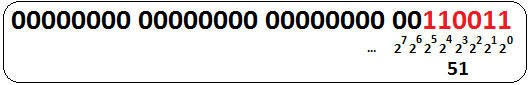``````// 110011
System.out.println("Binary: " + Integer.toString(51, 2));
System.out.println("Binary: " + Integer.toBinaryString(51));``````

``````System.out.println("Decimal: "
+ Integer.parseInt("110011", 2));  //51``````

## Bitwise operators

• 一元位补运算符[~]：作为一元，这个运算符需要放在数字之前的单个操作数。这个 运算符获取数字的每一位并将其值翻转，因此 1 变为 0，反之亦然；例如，5 = 101，~5 = 010。
• 按位与 [&]：此运算符需要两个操作数，并放置在两个数字之间。此运算符 逐一比较两个数字 的位。它充当逻辑 AND (&&)，这意味着它仅在比较位等于 1 时才返回 1；例如，5 = 101, 7 = 111, 5 & 7 = 101 & 111 = 101 = 5。
• 按位或 [|]：此运算符 需要两个操作数并放置在两个 数字之间。该运算符将两个数字的 位一一进行比较。它充当逻辑 OR (||)，这意味着如果至少有一个比较位为 1（或两者），则返回 1。否则，返回 0；例如，5 = 101, 7 = 111, 5 | 7 = 101 | 111 = 111 = 7。
• 按位异或 (XOR) [^]：此运算符 需要两个操作数，放在两个数字之间。此运算符 将两个数字的位一一进行比较。仅当比较的位具有不同的值时，它才返回 1。否则，返回 0；例如，5 = 101, 7 = 111, 5 ^ 7 = 101 | 111 = 010 = 2。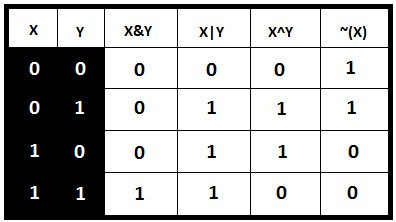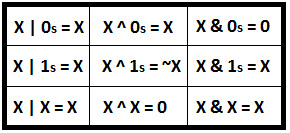## Bit shift operators

### Signed Left Shift [<<]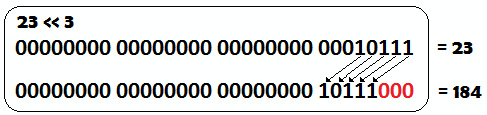1.将数字左移n个位置相当于乘以2n（例如，23 << 3等于 184，相当于 184 = 23 * 23)。

2.要移位的位置数自动减少到模32；即，23 << 35等价于23<< (35 % 32)，相当于 23 << 3.

### Signed Right Shift [>>]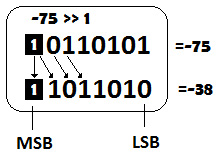（有符号的）二进制项中的所有 1 的序列以十进制形式表示 -1。

### Unsigned Right Shift [>>>]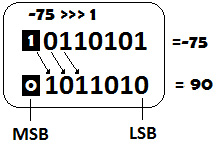## Tips and tricks

• 如果我们将一个数与自身 XOR[^] 偶数次，则结果为 0 (x ^ x = 0; x ^ x ^ x^ x = (x ^ x) ^ ( x ^ x) = 0 ^ 0 = 0)。
• 如果我们将一个数字与自身 XOR[^] 奇数次，那么结果就是那个数字 (x ^ x ^ x = (x ^ (x ^ x)) = (x ^ < /em>0) = x; x ^ x ^ x ^ x ^ x = (x ^ (x ^ x) ^ (x ^ x)) = (x ^ 0 ^ 0) = x)。
• 我们可以用 p > 计算表达式 p % q 的值； 0、q> 0，其中 q 是 2 的幂；即p& （q - 1）。 ComputeModuloDivision 是一个简单的应用程序，您可以在其中看到这一点。
• 对于给定的正整数 p，如果 ((p & 1) != 0) 和即使 ((p & 1) == 0)。 OddEven 是一个简单的应用程序，您可以在其中看到这一点。
• 对于两个给定的数字pq，我们可以说p 等于 q if ((p ^ q) == 0)。 CheckEquality 是一个简单的应用程序，您可以在其中看到这一点。
• 对于两个给定的整数 pq，我们可以通过 p 交换它们= p ^ q ^ (q = p)。 SwapTwoIntegers 是一个简单的应用程序，您可以在其中看到这一点。

# Coding challenges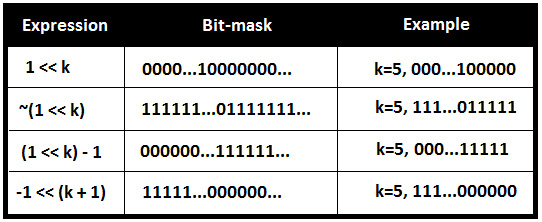## Coding challenge 1 – Getting the bit value``````public static char getValue(int n, int k) {
int result = n & (1 << k);
if (result == 0) {
return '0';
}
return '1';
}``````

## Coding challenge 2 – Setting the bit value``````public static int setValueTo0(int n, int k) {
return n & ~(1 << k);
}``````

1 | 1 = 1，而在 XOR[^] 运算符的情况下，我们写成 1 ^ 1 = 0。``````public static int setValueTo1(int n, int k) {
return n | (1 << k);
}``````

## Coding challenge 3 – Clearing bits``````public static int clearFromMsb(int n, int k) {
return n & ((1 << k) - 1);
}``````

``````public static int clearFromPosition(int n, int k) {
return n & ~((1 << k) - 1);
}``````

## Coding challenge 4 – Summing binaries on paper

1. 对当前列的所有位求和（第一列是 LSB 的列）。
2. 将结果转换为二进制（例如，通过连续除以 2）。
3. 保留最右边的位作为结果。
4. 将剩余位携带到其余列中（每列一位）。
5. 转到下一列并从 步骤 1 开始重复。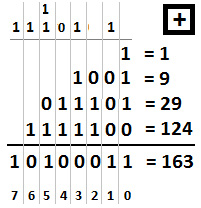• 第 0 列的总和位：1 + 1 + 1 + 0 = 3 = 11 1
• 第 1 列的总和位：1 + 0 + 0 + 0 = 1 = 1 1
• 第 2 列的总和位：0 + 1 + 1 = 2 = 10 0
• 第 3 列的总和位：1 + 1 + 1 + 1 = 4 = 100 0
• 第 4 列的总和位：0 + 1 + 1 = 2 = 10 0
• 第 5 列的总和位：1 + 1 + 0+1 = 3 = 1< /强>1 1
• 第 6 列的总和位：1 + 1 = 2 = 10 0
• 第 7 列的总和：1 = 1 = 1 1

## Coding challenge 5 – Summing binaries in code``````public static int sum(int q, int p) {
int xor;
int and;
int t;
and = q & p;
xor = q ^ p;
// force 'and' to return 0
while (and != 0) {
and = and << 1; // this is multiplication by 2
// prepare the next step of recursion
t = xor ^ and;
and = and & xor;
xor = t;
}
return xor;
}``````

## Coding challenge 6 – Multiplying binaries on paper

1. 从最右边的列（第 0 列）开始，将第二个二进制数的每一位乘以第一个二进制数的每一位。
2. 总结结果。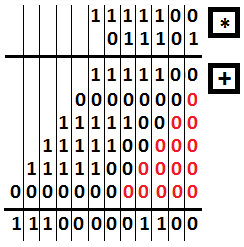## Coding challenge 7 – Multiplying binaries in code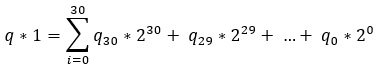1. 如果 p 的 LSB 为 1，那么我们写如下：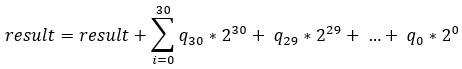图9.15 - p的LSB为1

2. 我们将 q 左移一位，逻辑右移 p 一位。
3. 我们从 step 1 开始重复，直到 p 为 0。

``````public static int multiply(int q, int p) {
int result = 0;
while (p != 0) {
// we compute the value of q only when the LSB of p is 1
if ((p & 1) != 0) {
result = result + q;
}
q = q << 1;  // q is left shifted with 1 position
p = p >>> 1; // p is logical right shifted with 1 position
}
return result;
}``````

## Coding challenge 8 – Subtracting binaries on paper

1. 从当前列中，我们搜索左列，直到找到一点 1。
2. 我们借用这一位并将其作为两个值 1 放在上一列中。
3. 然后，我们从前一列借用这两个值 1 中的一个作为其他两个 1。
4. 对每一列重复第 3 步，直到我们到达当前列。
5. 现在，我们可以执行计算了。
6. 如果我们遇到另一个 0 减 1，那么我们从 step 1 开始重复这个过程。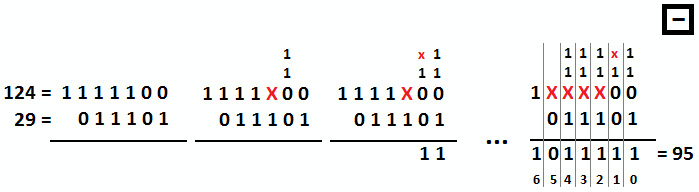1. 列 0 开始，所以从 0 减去 1。我们在左列中搜索，直到找到一点 1。我们在第 2 列找到它（该位对应到 22=4)。我们在第 1 列中借用该位并将其用作 1 的两个值（换句话说，2 中的两个是 21+21< /跨度>）。我们在第 0 列中借用这两个 1 值之一（这是 21=2）并将它们用作另外两个 1 值（换句话说，两个 1是 20+20)。现在，我们可以进行计算，因为 2 减 1 等于 1。我们记下 1 并移至第 1 列。
2. 我们继续第 1 列，因此 1 减去 0 等于 1。我们记下 1 并移至第 2 列。
3. 然后我们继续第 2 列，因此 0 减去 1。我们在左列中搜索，直到找到一点 1。我们在第 3 列找到它（该位对应于 2 3=8)。我们从第 2 列借用这个位并将其用作 1 的两个值（换句话说，2 中的两个是 22+22< /跨度>）。现在，我们可以进行计算，因为 2 减 1 等于 1。我们记下 1 并移至第 3 列。
4. 我们继续第 3 列，所以用 0 减去 1。我们在左列中搜索，直到找到一点 1。我们在第 4 列找到它（该位对应于 24 =16）。我们在第 3 列中借用这个位并将其用作 1 的两个值（换句话说，2 中的两个是 23+23< /跨度>）。现在，我们可以进行计算，因为 2 减 1 等于 1。我们记下 1 并移至第 4 列。
5. 我们继续第 4 列，所以用 0 减去 1。我们在左列中搜索，直到找到一点 1。我们在第 5 列找到它（该位对应于 25 =32）。我们在第 4 列中借用这个 位并将其用作两个值 1（换句话说，2 中的两个是 24 +24）。现在，我们可以进行计算，因为 2 减 1 等于 1。我们记下 1 并移至第 5 列。
6. 我们继续第 5 列，所以用 0 减去 0。我们记下 0 并移至第 6 列。
7. 我们继续第 6 列，所以用 1 减去 0。我们写下 1，然后我们就完成了。

## Coding challenge 9 – Subtracting binaries in code

|1 - 1| = 1 ^ 1 = 0, |1 - 0| = 1 ^ 0 = 1, |0 - 1| = 0 ^ 1 = 1 和 |0 - 0| = 0 ^ 0 = 0。

``````public static int subtract(int q, int p) {
while (p != 0) {
// borrow the unset bits of q AND set bits of p
int borrow = (~q) & p;
// subtraction of bits of q and p
// where at least one of the bits is not set
q = q ^ p;
// left shift borrow by one position
p = borrow << 1;
}
return q;
}``````

## Coding challenge 10 – Dividing binaries on paper

a.a) 我们将股息的下一位附加到子股息并从 步骤 a 继续。

b.a) 我们从当前的子股息中减去 除数。

b.b) 我们将下一位股息附加到减法的结果（这是新的子股息），然后从 step a).

C。当我们处理完被除数的所有位后，我们应该得到商和余数，这是除法的结果。

c.a) 我们可以在这里停下来，用得到的商和余数来表达结果。

c.b) 我们可以将一个点 (".") 附加到商上，并将 0 附加到当前余数（这是新的子股息），然后从 step a 直到余数为 0 或者我们对结果感到满意。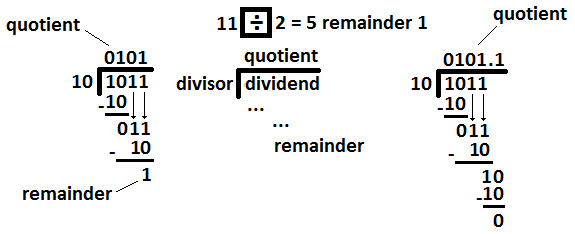• 分红 = 1, 10 > 1 自 2 > 1，因此我们将 0 附加到商。
• 分红 = 10, 10 = 10 因为 2 = 2，因此我们将 1 附加到商。
• 做减法，10 - 10 = 0。
• 分红 = 01, 10 > 01 自 2 > 1，因此我们将 0 附加到商。
• 分红 = 011, 10 < 011 自 2 ＜ 3，因此我们将 1 附加到商。
• 做减法，011 - 10 = 1。
• 从被除数中处理没有更多位，因此我们可以说11/2的商为101（即5），余数为1。

## Coding challenge 11 – Dividing binaries in code

``````private static final int MAX_BIT = 31;
...
public static long divideWithoutRemainder(long q, long p) {
// obtain the sign of the division
long sign = ((q < 0) ^ (p < 0)) ? -1 : 1;
// ensure that q and p are positive
q = Math.abs(q);
p = Math.abs(p);
long t = 0;
long quotient = 0;
for (int i = MAX_BIT; i >= 0; --i) {
long halfdown = t + (p << i);
if (halfdown <= q) {
t = t + p << i;
quotient = quotient | 1L << i;
}
}
return sign * quotient;
}``````

## Coding challenge 12 – Replacing bits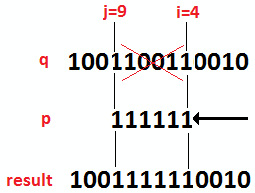1110000000000位掩码可以通过-1左移j+1个位置得到，而0000000001111位掩码可以通过左移1i 个位置并减去 1。``````public static int replace(int q, int p, int i, int j) {
int ones = ~0; // 11111111 11111111 11111111 11111111
int leftShiftJ = ones << (j + 1);
int leftShiftI = ((1 << i) - 1);
int mask = leftShiftJ | leftShiftI;
int bringPInPlace = p << i;
}``````

## Coding challenge 13 – Longest sequence of 1• 初始化最长序列 = 0。
• 应用 AND[&]:10000011111001110 & 1 = 0，最长序列 = 0。
• 右移并应用 AND[&]:1000001111100111 & 1 = 1，最长序列 = 1。
• 右移并应用 AND[&]:100000111110011 & 1 = 1，最长序列 = 2。
• 右移并应用 AND[&]:10000011111001 & 1 = 1，最长序列 = 3。
• 右移并应用 AND[&]:1000001111100 & 1 = 0，最长序列 = 0
• 右移并应用 AND[&]:100000111110 & 1 = 0，最长序列 = 0。
• 右移并应用 AND[&]:10000011111 & 1 = 1，最长序列 = 1。
• 右移并应用 AND[&]:1000001111 & 1 = 1，最长序列 = 2。
• 右移并应用 AND[&]:100000111 & 1 = 1，最长序列 = 3。
• 右移并应用 AND[&]:10000011 & 1 = 1，最长序列 = 4。
• 右移并应用 AND[&]:1000001 & 1 = 1，最长序列 = 5。
• 右移并应用 AND[&]:100000 & 1 = 0，最长序列 = 0。

• 检查下一位是 1 还是 0，(n & 2) == 1 或 0
• 如果下一位为 1，则检查前一位是否为 1

``````public static int sequence(int n) {
if (~n == 0) {
return Integer.SIZE; // 32
}
int currentSequence = 0;
int longestSequence = 0;
boolean flag = true;
while (n != 0) {
if ((n & 1) == 1) {
currentSequence++;
flag = false;
} else if ((n & 1) == 0) {
currentSequence = ((n & 0b10) == 0) // 0b10 = 2
? 0 : flag
? 0 : ++currentSequence;
flag = true;
}
longestSequence = Math.max(
currentSequence, longestSequence);
n >>>= 1;
}
return longestSequence;
}``````

## Coding challenge 14 – Next and previous numbers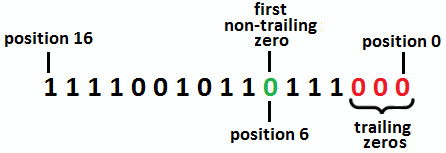11110010111011000124376 > 11110010111000011124355

``````int copyn = n;
int zeros = 0;
while ((copyn != 0) && ((copyn & 1) == 0)) {
zeros++;
copyn = copyn >> 1;
}``````

``````int ones=0;
while ((copyn & 1) == 1) {
ones++;
copyn = copyn >> 1;
}``````

``n = n | (1 << marker);````n = n & (-1 << marker);````n = n | (1 << (ones - 1)) - 1;````````public static int next(int n) {
int copyn = n;
int zeros = 0;
int ones = 0;
// count trailing 0s
while ((copyn != 0) && ((copyn & 1) == 0)) {
zeros++;
copyn = copyn >> 1;
}
// count all 1s until first 0
while ((copyn & 1) == 1) {
ones++;
copyn = copyn >> 1;
}
// the 1111...000... is the biggest number
if (zeros + ones == 0 || zeros + ones == 31) {
return -1;
}
int marker = zeros + ones;
n = n | (1 << marker);
n = n & (-1 << marker);
n = n | (1 << (ones - 1)) - 1;
return n;
}``````

## Coding challenge 15 – Conversion

Solution：如果我们观察到 XOR[^] 运算符仅在操作数不同时返回 1，那么这个问题的解决方案就很清楚了。让我们考虑 q = 290932 (1000111000001110100) 和 p = 352345 (1010110000001011001)。让我们应用 XOR[^] 运算符：``````public static int count(int q, int p) {
int count = 0;
// each 1 represents a bit that is
// different between q and p
int xor = q ^ p;
while (xor != 0) {
count += xor & 1; // only 1 & 1 = 1
xor = xor >> 1;
}
return count;
}``````

## Coding challenge 16 – Maximizing expressions

a = 1, 1 * 4 = 4

a = 2, 2 * 4 = 8

a = 3, 3 * 4 = 12

a = 4, 4 * 4 = 16(1 AND s) = (0 AND s) → s = 0 → (1 & 0) = (0 & 0) = 0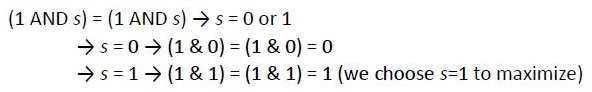## Coding challenge 17 – Swapping odd and even bits

1. 我们取奇数位并将它们向右移动一个位置。
2. 我们取偶数位并将它们向左移动一个位置。``````public static int swap(int n) {
int moveToEvenPositions
= (n & 0b10101010101010101010101010101010) >>> 1;
int moveToOddPositions
= (n & 0b1010101010101010101010101010101) << 1;
return moveToEvenPositions | moveToOddPositions;
}``````

## Coding challenge 18 – Rotating bits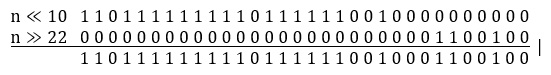``````public static int leftRotate(int n, int bits) {
int fallBits = n << bits;
int fallBitsShiftToRight = n >> (MAX_INT_BITS - bits);
return fallBits | fallBitsShiftToRight;
}``````

``````public static int rightRotate(int n, int bits) {
int fallBits = n >> bits;
int fallBitsShiftToLeft = n << (MAX_INT_BITS - bits);
return fallBits | fallBitsShiftToLeft;
}``````

## Coding challenge 19 – Calculating numbers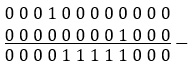``````public static int setBetween(int left, int right) {
return (1 << (right + 1)) - (1 << left);
}``````

## Coding challenge 20 – Unique elements

• 第一位的总和 % 3 = 0+0+1+1+1+1+1+1+1+0 = 7 % 3 = 1
• 第二位的总和 % 3 = 0+0+1+0+1+1+1+0+0+0 = 4 % 3 = 1
• 第三位之和 % 3 = 1+1+0+0+1+1+1+0+0+1 = 6 % 3 = 0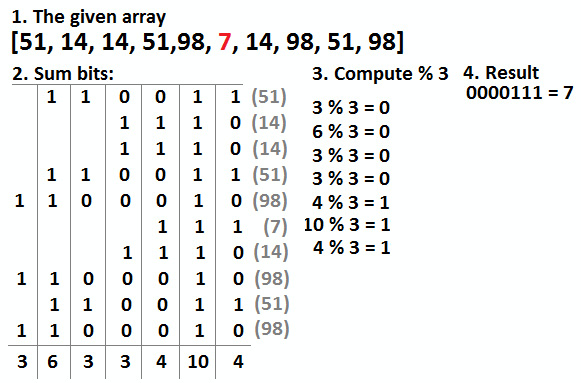1. 总结 相同位置的位。
2. 对于每个 sum，计算模数 3。
3. 如果 sum % 3 = 0（sum 是 3 的倍数），这意味着该位在元素中设置在给定的元素中出现三次。
4. 如果 sum % 3 ! = 0（sum 不是 3 的倍数），这意味着该位在出现一次的元素中设置（但不是确定该位是否未设置或在出现三次的元素中设置）。
5. 我们必须重复 步骤 123给定元素和位的所有位置。通过执行此操作，我们将获得仅出现一次的元素，正如您在上图中看到的那样。

``````private static final int INT_SIZE = 32;
public static int unique(int arr[]) {
int n = arr.length;
int result = 0;
int nr;
int sumBits;
// iterate through every bit
for (int i = 0; i < INT_SIZE; i++) {
// compute the sum of set bits at
// ith position in all array
sumBits = 0;
nr = (1 << i);
for (int j = 0; j < n; j++) {
if ((arr[j] & nr) == 0) {
sumBits++;
}
}
// the sum not multiple of 3 are the
// bits of the unique number
if ((sumBits % 3) == 0) {
result = result | nr;
}
}
return result;
}``````

1. 对于每个新元素，将它的 XOR[^] 放在一个变量中，`oneAppearance`
2. 如果元素第二次出现，那么它将从 `oneAppearance` 中删除，我们将它的 XOR[^] 放在另一个变量中，```twoAppearances 。```
3. 如果该元素第三次出现，那么它将从 `oneAppearance``twoAppearances` 中删除。 `oneAppearance``twoAppearances` 变量变为 0，我们开始寻找新元素。
4. 对于所有出现 3 次的元素，`oneAppearance``twoAppearances` 变量将为 0。另一方面，对于元素仅出现一次，`oneAppearance` 变量将设置为 该值。

``````public static int unique(int arr[]) {
int oneAppearance = 0;
int twoAppearances = 0;
for (int i = 0; i < arr.length; i++) {
twoAppearances = twoAppearances
| (oneAppearance & arr[i]);
oneAppearance = oneAppearance ^ arr[i];
int neutraliser = ~(oneAppearance & twoAppearances);
oneAppearance = oneAppearance & neutraliser;
twoAppearances = twoAppearances & neutraliser;
}
return oneAppearance;
}``````

## Coding challenge 21 – Finding duplicates

``````  private static final int MAX_N = 32000;
public static void printDuplicates(int[] arr) {
BitSet bitArr = new BitSet(MAX_N);
for (int i = 0; i < arr.length; i++) {
int nr = arr[i];
if (bitArr.get(nr)) {
System.out.println("Duplicate: " + nr);
} else {
bitArr.set(nr);
}
}
}``````

## Coding challenge 22 – Two non-repeating elements

• 如果我们将一个数与自身异或[^]偶数次，则结果如下 0 (x ^ x = 0; x ^ x ^ x^ x = (x ^ x) ^ ( x ^ x) = 0 ^ 0 = 0)

xor = 2 ^ 7 ^ 1 ^ 5 ^ 9 ^ 4 ^ 1 ^ 2 ^ 5 ^ 4 = 0010 (2) ^ 0111 (7) ^ 0001 (1) ^ 0101 (5) ^ 1001 (9) ^ 0100 (4) ^ 0001 (1) ^ 0010 (2) ^ 0101 (5) ^ 0100 (4) = 1110 = 7 ^ 9 = 0111 & 1001 = 1110 = 14。

xor_second_set = 1 ^ 5 ^ 9 ^ 4 ^ 1 ^ 5 ^ 4 = 0001 ^ 0101 ^ 1001 ^ 0100 ^ 0001 ^ 0101 ^ 0100 = 1001 = 9（第二个非重复元素）。

``````public static void findNonRepeatable(int arr[]) {
// get the XOR[^] of all elements in the given array
int xor = arr;
for (int i = 1; i < arr.length; i++) {
xor ^= arr[i];
}
// get the rightmost set bit (you can use any other set bit)
int setBitNo = xor & ~(xor - 1);
// divide the elements in two sets by comparing the
// rightmost set bit of XOR[^] with the bit at the same
// position in each element
int p = 0;
int q = 0;
for (int i = 0; i < arr.length; i++) {
if ((arr[i] & setBitNo) != 0) {
// xor of the first set
p = p ^ arr[i];
} else {
// xor of the second set
q = q ^ arr[i];
}
}
System.out.println("The numbers are: " + p + " and " + q);
}``````

## Coding challenge 23 – Power set of a set

20=000, 21=001, 22=010, 23=011, 24=100, 25=101, 26=110, 27=111

20 = 000 = {}

21 = 001 = {a}

22 = 010 = {b}

23 = 011 = {a, b}

24 = 100 = {c}

25 = 101 = {a, c}

26 = 110 = {b, c}

27 = 111 = {a, b, c}

``````Compute the Power Set size as 2 size of S
Iterate via i from 0 to Power Set size
Iterate via j from 0 to size of S
If jth bit in i is set then
Add jth element from set to current subset
Add the resulted subset to subsets
Return all subsets``````

``````public static Set<Set<Character>> powerSet(char[] set) {
// total number of subsets (2^n)
long subsetsNo = (long) Math.pow(2, set.length);
// store subsets
Set<Set<Character>> subsets = new HashSet<>();
// generate each subset one by one
for (int i = 0; i < subsetsNo; i++) {
Set<Character> subset = new HashSet<>();
// check every bit of i
for (int j = 0; j < set.length; j++) {
// if j'th bit of i is set,
// add set[j] to the current subset
if ((i & (1 << j)) != 0) {
}
}
}
return subsets;
}``````

## Coding challenge 24 – Finding the position of the only set bit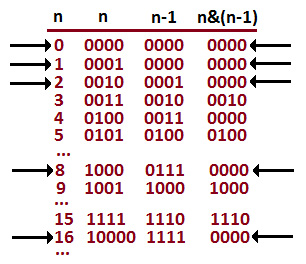``````public static int findPosition(int n) {
int count = 0;
if (!isPowerOfTwo(n)) {
return -1;
}
while (n != 0) {
n = n >> 1;
++count;
}
return count;
}
private static boolean isPowerOfTwo(int n) {
return (n > 0) && ((n & (n - 1)) == 0);
}``````

## Coding challenge 25 – Converting a float into binary and vice versa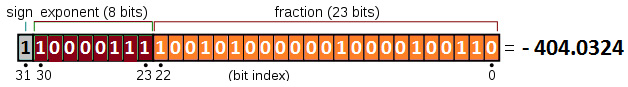`float` 值，当由具有给定符号、有偏指数、e 的 32 位二进制数据表示时，（8位无符号整数）和一个 23 位小数，如下所示：